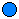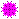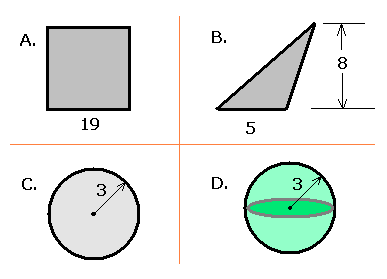### MS 095 PreAlgebra Test 01 • Name: __________

#### Matching

Marble:Pompom:____sulp=sulpA. Additive Inverse Property ____sulp= [Nothing] B. Commutative Property ____sulp nothing =C. Additive property of zero
1. ____________________ What is 0 ÷ 0 equal to?
2. ____________________ What is÷ 0 equal to?
3. ____________________ What is 0 ÷ 42 equal to?
4. ____________________ What issunimequal to?
5. Identify the constants and variables in: y = 7e3x
6. __________ Calculate 194
7. __________ Calculate 2p2
8. __________ Calculate e2
9. __________ What is 10016 ÷ 2?
10. __________ What is the largest single digit “number” in base 9?
11. __________ What color light results from adding red light to blue light on the computer monitor?
12. The following data is the seat count for the seats in the FSM-China Friendship Sports Center as reported on quiz one:

1169, 1209, 1219, 1224, 1229, 1260, 1290, 1291, 1291, 1363, 1446, 1466

1. ______ What is the mode for the seat count data?
2. ______ What is the median for the seat count data?
3. ______ What is the mean (average) for the seat count data?
13. For the following diagram:1. __________ What is the perimeter of the square with one side 19 cm in length shown in A?
2. __________ What is the area of the square with one side 19 cm in length shown in A?
3. __________ What is the area of the triangle with base 5 cm and height 8 cm shown in B?
4. __________ What is the perimeter of the circle with radius 3 meters shown in C?
5. __________ What is the area of the circle with radius 3 meters shown in C?
6. __________ What is the volume of the sphere with radius 3 meters shown in D?